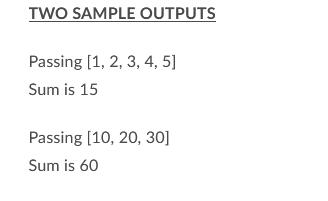# TWO SAMPLE OUTPUTSPassing [1, 2, 3, 4, 5]Sum is 15Passing [10, 20, 30]Sum is 60

Question
14 views

Write a method named sumInts that can take a variable number of int arguments and return the sum of these arguments. The ints to be summed up must be entered as command line arguments. Command line arguments can be simulated in Eclipse. In the main method, display the ints that were entered on the command line. Then execute sumInts and display the sum it returns.help_outlineImage TranscriptioncloseTWO SAMPLE OUTPUTS Passing [1, 2, 3, 4, 5] Sum is 15 Passing [10, 20, 30] Sum is 60 fullscreen
check_circle

Step 1

Program Approach:

• Import the essential Java Packages.
• Define the Main method.
• Define the sumInts method.
• Argument will be provided in the format of integer array type.
• Use If-Else statement to check the condition.
• Check for the variables whether they are provided as Command-Line arguments or not.
• Create an array in the If statement, and a For loop for storing the Command-Line arguments in the num array.
• Initialize the variables as per the usage in the sumInts method.
• Use for loop to calculate the value of variable total.
• Display the result on the console.

Note: Arguments which are passed in the sumInts() function are taken as command-line arguments.

Step 2

Code(in java)-

//Import the essential package

import java.util.*;

// Declaring class

class Main

{

//defining main method

public static void main(String[] a)

{

//To determine whether, there are variable arguments in the command line or not

if (a.length!=0)

{

//Create the object of an array

int[] number = new int[a.length];

for (int i = 0; i < number.length; i++)

{

//command line arguments into integer type is converted use typecasting

//Store variables in number array

number[i] = Integer.parseInt(a[i]);

}

//Dsiplaying the result

System.out.println("Executing " + Arrays.toString(number));

System.out.println("The sum is " + sumInts(number));

}

else

{...

### Want to see the full answer?

See Solution

#### Want to see this answer and more?

Solutions are written by subject experts who are available 24/7. Questions are typically answered within 1 hour.*

See Solution
*Response times may vary by subject and question.
Tagged in

### Computer Engineering